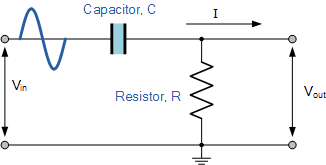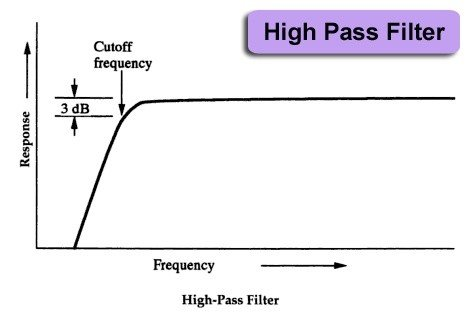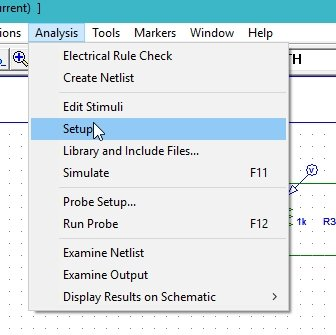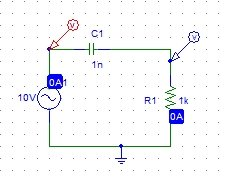# High pass filter simulation using PSpice : tutorial 14

In this tutorial I will explain you the working of a high pass filter. The purpose of a low pass filter is to allow only the higher frequencies to pass through the circuit and block the lower frequencies. At the start a brief and concise introduction of filters specifically high pass filter along with them bode plots are provided with the explanation of the output they will show. After that the circuits is simulated using PSPICE and you are provided with the step by step approach to simulate a circuit and the result are compared with the theoretical discussion provided (which should be same). At the end of the tutorial you are provided with an exercise to do it by yourself, and in the next tutorials I will assume that you have done those exercises and I will not explain the concept regarding them

### Introduction to high pass filter

A high pass filter is such a filter which only allows frequencies with high magnitude to pass through them and block the lower frequencies as the name suggests. As simple high pass filter is shown in the figure below.Figure 1: High pass filter

The limit of the frequencies after which the circuit allows is known as critical frequency and is given by the formula,

f = 1 / 2piRC

This frequency is present at -3db of the maximum magnitude or 0.707 or 70% of the maximum magnitude. The output plot in frequency domain or the bode plots of a simple high pass filter is given in the figure below,Figure 2: High pass filter

Simulation of HIGH Pass filter using PSpice

Figure 3: Opening schematic

Figure 4: Saving schematic

Figure 5: getting new part

Figure 6: Placing ac input

Figure 7: Placing capacitor

Figure 8: Placing resistor

Figure 9: Placing ground

Figure 10: Placed components

Figure 11: Draw wire

Figure 12: Complete circuit diagram

Figure 13: Voltage marker

Figure 14: Voltage marker placed

Figure 15: Input source attributes

• The adjustable attributes in the AC supply is only the magnitude of the AC voltage and all other attributes should be left as it is. The DC attribute is used to adjust the offset of the ac voltage which is not need in this experiment hence left as 0.
• Set the value of the capacitor according to the requirement of your circuit by double clicking on the component and changing the value to 3u and then clicking save attribute but I am leaving the value in this experiment as it is.
• Next step is to adjust the properties of the simulations in order to produce the graph of the voltage at the marker. Click on analysis and then click on Setup as shown in the figure belowFigure 16: Simulation setup

Figure 17: AC sweep properties

Figure 18: Labeling the wire

Figure 19: Simulation

Figure 20: Output of simulation

• From the output it is obvious that the circuit simulated is a high pass filter. Above is shown the bode plot (frequency domain plot) of the circuit. At higher frequencies the circuit is allowing the voltage to pass through it and at lowerer frequencies it drops down to zero. Also the results are in accordance with the theoretical introduction.
• Connect another voltage marker at the input of the AC source to the see the AC sweep of the source along with that of the capacitor as shown in the figure below,Figure 21: Voltage maker at input

Figure 22: Output displayed with input

• The green line at the top represents the input AC sweep of the AC source connect. The straight line represents that the input source is constant (neither increasing nor decreasing with time) unlike that of the capacitor voltage. In the frequency domain we can say that the input source is allowing all the frequencies to pass hence straight.

Exercise:

• Try do the frequency domain analysis of any of the circuits we have done previously.

### 1 thought on “High pass filter simulation using PSpice : tutorial 14”

1. Did you state this backwards? “The purpose of a low pass filter is to allow only the higher frequencies to pass through the circuit and block the lower frequencies.”

You stated it correctly in the next paragraph. “A high pass filter is such a filter which only allows frequencies with high magnitude to pass through them and block the lower frequencies as the name suggests.”